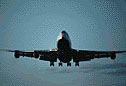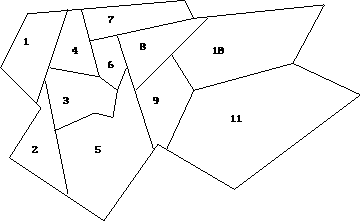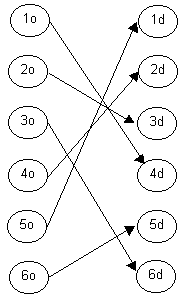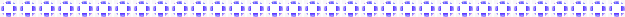3.0 Core ProblemIn this problem, we will integrate the set covering and transportation problems to solve the optimal flight plan problem. We are given an area to search as shown in the figure below. The entire area is divided into 11 smaller regions. We need to model the figure as a set covering problem to find which regions the plane should be so that the camera can cover the whole area. Then, we take the optimal solution and find the best route to travel within those regions.3.1 Problem Statement (Set Covering)

Model the graph below as a set covering problem to find the optimal area where the plane should be. Assume that the camera is moving at 3600 and cover the area below it and the surrounding areas. For example, if the camera is above 3, it can scan all the areas 1, 2, 3, 4, 5, 6.Model

 Sets i - small areas numbered 1 to 11 Variables Xi = 1 if the plane is in area I XI = 0 otherwise Objective Function Z = S Xi (for i = 1 to 11)

Solution Summary

The optimal value is Z = 3

The optimal solution is X3 = 1, X8 = 1, X9 = 1, Xi = 0 where i = 1,2,4,5,6,7,10,11

This implies that if the plane is above area 3, area 8, and area 9, it will cover the whole searched area.

3.2 Problem Statement (Transportation)

Given the optimal solution from the set covering model, the objective of this problem is to find the optimal flight plan ( ie minimum cost flight plan) to each of the nodes designated by the set covering model.

Model

 Sets I - areas from which we travel to next area to be searched {3o, 5o, 6o, 8o, 9o} J - areas to be searched {3d, 5d, 6d, 8d, 9d} Variables X(I,J) - represents travelling on path i-j Z - total search cost in dollars Objective Function min S iE IS jE J CijXij

Solution Summary

The objective value is 13.

Pictorial Optimal Flight Plan: